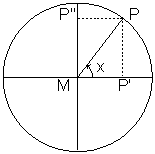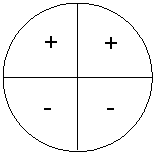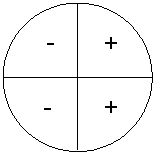# Unit circle and simple formulas

## Summary and examples

We will define the following three standard functions from trigonometry: $(\sin(x)$, $\cos(x)$ and $\tan(x))$. We do this by using a right-angled triangle $ABC$. We call the angle $BAC$ $x$, see the figure:

 $\sin(x)=\displaystyle\frac{BC}{AC}$ $\cos(x)=\displaystyle\frac{AB}{AC}$ $\tan(x)=\displaystyle\frac{BC}{AB}$$x$ is the angle either in degrees or radians (see later for this).
In the figure the angle is an acute angle $x$, so $0\leq{x}\leq{90}$. When the angle is outside this interval these definitions cannot be used. That is why we use the unit circle, i.e. a circle with center $(0,0)$ and radius $1$. Now we see that $x$ is equal to the angle $PMP'$.

 $\sin(x)=\displaystyle\frac{PP'}{MP}=PP'=MP''$ $\cos(x)=\displaystyle\frac{MP'}{MP}=MP'$Now $\sin(x)$ can be defined as the projection of $P$ on the $Y$-axis and $\cos(x)$ as the projection of $P$ on the $X$-axis. These definitions allow us to define $\sin(x)$ and $\cos(x)$ functions also for other angles than acute angles. In the figures below various angles are shown with $P$ in the second, third and fourth quadrant, respectively.

We see that $\sin(x)=MP''$ is positive, negative, negative, respectively. For $cos(x)=MP'$ we have negative, negative, positive, respectively.

We can show this in the following figures:

 $\sin(x)$$\sin(x)$ is positive in the first and second quadrant, and negative in the third and fourth quadrant. $\cos(x)$$\cos(x)$ is positive in the first and fourth quadrant and negative in the second and third quadrant.

From these figures we can deduce some simple and well-known formulas.

$\sin(-x)=-\sin(x)$

$\cos(-x)=\cos(x)$

Later we will show a few other simple formulas based on the unit circle, but first we will introduce the radian which is another unit for angles. The relationship between an angle in degrees and an angle in radians is:

360 degrees= $2\pi$ radians (in brief rads)

and thus we have:

1 rad = $\displaystyle\frac{360}{2\pi}\approx 57$ degrees

Following we will use radians unless mentioned otherwise. We will give some more formulas that can easily be deduced from the unit circle (sometimes we use congruent triangles).

$\sin(\displaystyle\frac{\pi}{2}+x)=\cos(x)$

$\sin(\displaystyle\frac{\pi}{2}-x)=\cos(x)$

To calculate the $\sin$ of an arbitrary angle $x$, we usually need a calculator (remember to put it in the radians mode when the angles are given in radians). For a few special angles, we use the following table:

 $0$ $\displaystyle\frac{\pi}{6}$ $\displaystyle\frac{\pi}{4}$ $\displaystyle\frac{\pi}{3}$ $\displaystyle\frac{\pi}{2}$ $\sin(x)$ $\displaystyle\frac{1}{2}\sqrt{0}$ $\displaystyle\frac{1}{2}\sqrt{1}$ $\displaystyle\frac{1}{2}\sqrt{2}$ $\displaystyle\frac{1}{2}\sqrt{3}$ $\displaystyle\frac{1}{2}\sqrt{4}$ $\cos(x)$ $\displaystyle\frac{1}{2}\sqrt{4}$ $\displaystyle\frac{1}{2}\sqrt{3}$ $\displaystyle\frac{1}{2}\sqrt{2}$ $\displaystyle\frac{1}{2}\sqrt{1}$ $\displaystyle\frac{1}{2}\sqrt{0}$ $\tan(x)$ $0$ $\displaystyle\frac{1}{3}\sqrt{3}$ $1$ $\sqrt{3}$ -

You can recall this table easily by remembering the first row (the $\sin(x)$-row), where the number under the root sign is subsequently $0, 1 ,2, 3$ and $4$. The $\cos(x)$-row can be used in a similar way, though from right to left. We get the $\tan(x)$-row by dividing the $\sin(x)$-row by the $\cos(x)$-row.
Now we have defined the $\sin$- and $\cos$-function, we can summarize a number of properties.

 $y=\sin(x)$ $x$ is a real number, $y$ lies in the interval $[-1,+1]$. This means that the amplitude (the maximum value of $|y|$ is equal to $1$. The period of this function is equal to $2\pi$, because after $2\pi$ radians the function repeats the same values. The function has zeros (is equal to $0$) if $x=k\pi$ with $k$ a whole number. The function has extremes for $x=(2k+1)\displaystyle\frac{\pi}{2}$ with $k=0, \pm1, \pm2, ...$ $y=\cos(x)$ $x$ is a real number, $y$ lies in the interval $[-1,+1]$. This means that the amplitude (the maximum value of $|y|$ is equal to $1$. The period of this function is equal to $2\pi$, because after $2\pi$ radians the function repeats the same values. The function has zeros for $x=(2k+1)\pi$ with $k=0, \pm1, \pm2, ...$. The function has extremes for $x=k\pi$, with $k=0, \pm1, \pm2, ...$

Earlier we mentioned a number of simple formulas. A few formulas occur so frequently that you can better learn them by heart:

$\sin^2(x)+\cos^2(x)=1$

$\tan(x)=\displaystyle\frac{\sin(x)}{\cos(x)}$

$\sin(2x)=2\sin(x)\cos(x)$

$\cos(2x)=\cos^2(x)-\sin^2(x)$

The latter formula can be written in another form by using the first formula:

$\cos(2x)=2\cos^2(x)-1$

$\cos(2x)=1-2\sin^2(x)$

The first two formulas can easily be deduced from earlier given definitions. We know that:

$\sin(x)=\displaystyle\frac{BC}{AC}$

$\cos(x)=\displaystyle\frac{AB}{AC}$

and thus:

$\sin^2(x)+\cos^2(x)=$

$=(\displaystyle\frac{BC}{AC})^2+(\displaystyle\frac{AB}{AC})^2=\displaystyle\frac{BC^2+AB^2}{AC^2}=\displaystyle\frac{AC^2}{AC^2}=1$

We used the Pythagoras' Theorem.

The second formula can also be deduced easily:

$\displaystyle\frac{\sin(x)}{\cos(x)}=\displaystyle\frac{\displaystyle\frac{BC}{AC}}{\displaystyle\frac{AB}{AC}}=\displaystyle\frac{BC}{AB}=\tan(x)$

Finally, there are a number of formulas that are less frequently used. Then it does not really pay to remember them heart. Sometimes they are necessary, but then a formula sheet can be used.

##### Example 1

Explain using the unit circle that the following formula holds (angle $x$ in degrees):

$\cos(68)=\sin(22)$

The angle $68$ degrees can be written as $68 = 90-22$ degrees.
The projection of $P$ with $68$ degrees on the $X$-axis is equal to the projection of $P$ with $22$ degrees on de $Y$-axis. This can be proven by congruence of triangles. So this relation is valid.

##### Example 2

Explain using the unit circle that the following formula holds (angle $x$ in radians):

$\cos(\displaystyle\frac{\pi}{2}-x)=\sin(x)$

This formula is the same as in example 1, though formulated more generally. Again, this can be proven using the congruence of triangles.

##### Example 3

Calculate without a calculator $\sin(225)$, the angle is given in degrees.
We can write the formula as $\sin(180+45)$ and thus the angle is in the third quadrant. We notice that $\sin(180+45) = -\sin(45)$ and this value can be found in the table:

$\sin(225)=-\displaystyle\frac{1}{2}\sqrt{2}$

##### Example 4

Prove:

$[\sin(x)+\cos(x)]^2=1+\sin(2x)$

The left-hand side can be written as:

$[\sin(x)+\cos(x)]^2=\sin^2(x)+2\sin(x)\cos(x)+\cos^2(x)$

We know that:

$\sin^2(x)+\cos^2(x)=1$

$2\sin(x)\cos(x)=\sin(2x)$

and thus the relation is proven.

0